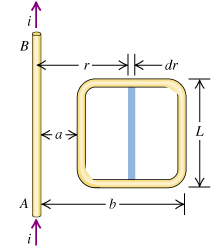# Problem: The current in the long, straight wire AB shown in the figure(Figure 1) is upward and is increasing steadily at a rate di/dt Evaluate the numerical value of the induced emf if a=12.0cm, b=36.0cm, L=24.0cm, and di/dt=9.60A/s

###### FREE Expert Solution

Induced emf:

$\overline{){\mathbf{\epsilon }}{\mathbf{=}}\frac{\mathbf{d}\mathbf{\varphi }}{\mathbf{d}\mathbf{t}}{\mathbf{=}}\frac{{\mathbf{\mu }}_{\mathbf{0}}\mathbf{L}}{\mathbf{2}\mathbf{\pi }}\frac{\mathbf{d}\mathbf{i}}{\mathbf{d}\mathbf{t}}{\mathbf{l}}{\mathbf{n}}{\mathbf{\left(}}\frac{\mathbf{b}}{\mathbf{a}}{\mathbf{\right)}}}$

81% (133 ratings)###### Problem Details

The current in the long, straight wire AB shown in the figure(Figure 1) is upward and is increasing steadily at a rate di/dtEvaluate the numerical value of the induced emf if a=12.0cm, b=36.0cm, L=24.0cm, and di/dt=9.60A/s